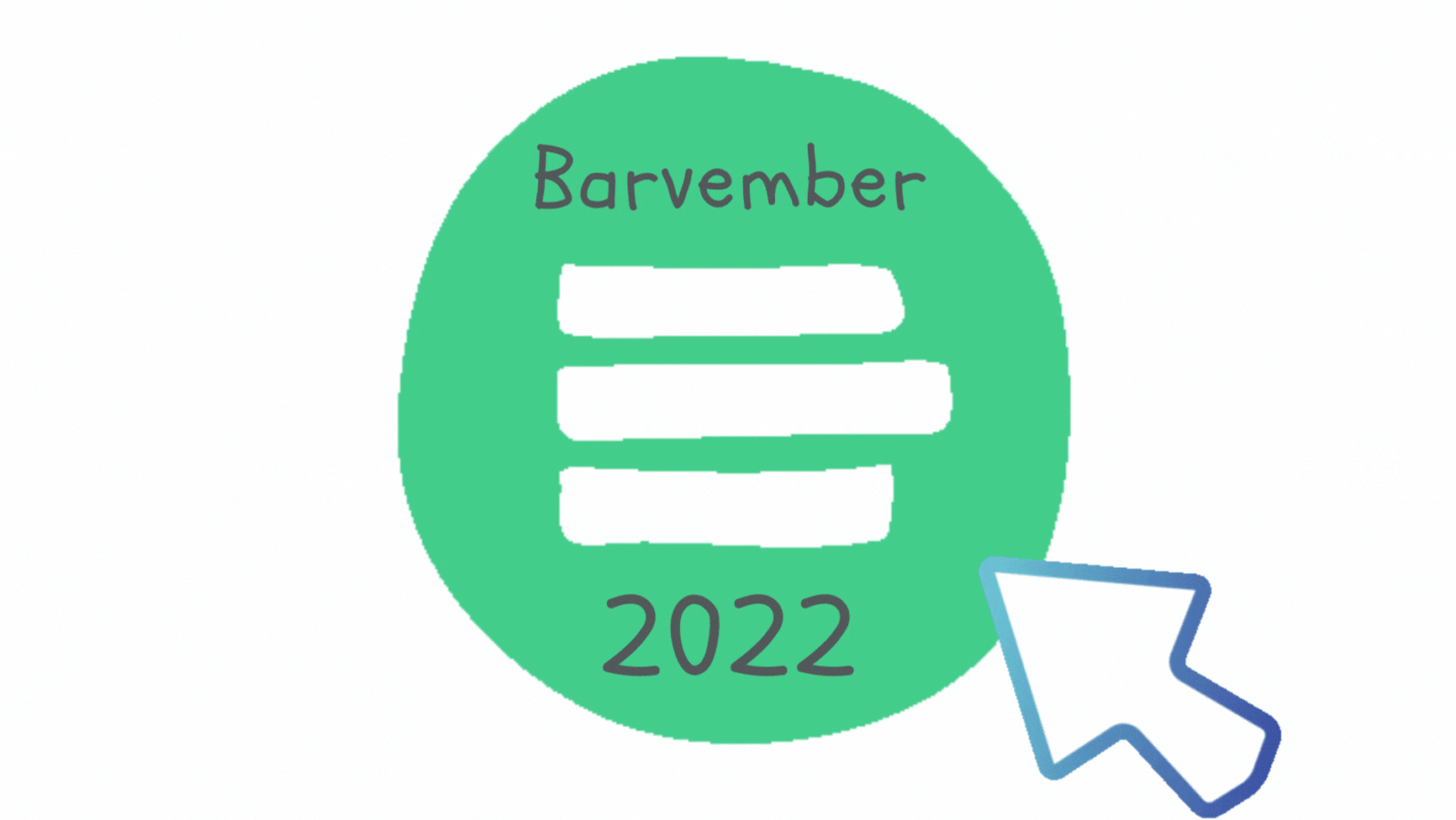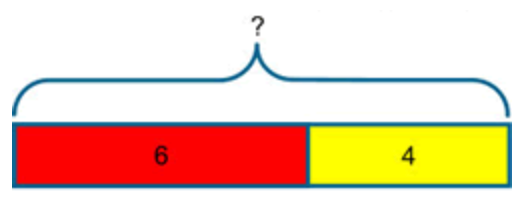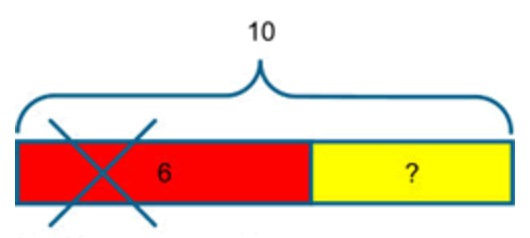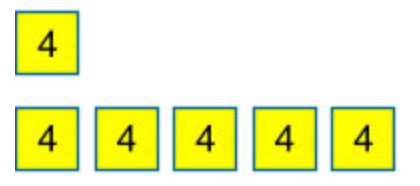top of page

# Barvember 2022

Barvember is back at ISB!Throughout the month of November, we will be taking part in a series of Maths challenges: 'Barvember'. Barvember makes reference to Bar Modelling. The bar model is used to support children in problem solving.

Each day throughout November, we will be posting a new set of challenges (and answers) in Seesaw. Questions increase in difficulty and we recommend discussing with your child to find the appropriate level, we do not expect all students to complete all of the questions.

Question 1 is aimed at our youngest students with Question 4 only appropriate for Yr5 and Yr6. This is optional additional home learning and a great way for children to teach parents how they solve Maths problems in school. Completed answers can be posted back to Seesaw, either as a photo or a video of the family working together to solve the challenges.

Good luck and enjoy 'Barvember'!## 🔎 Find out even more...

The bar model is used in teaching for mastery to help children to 'see' mathematical structure.

It is not a method for solving problems, but a way of revealing the mathematical structure within a problem and gaining insight and clarity to help solve it. It supports the transformation of real-life problems into a mathematical form and can bridge the gap between concrete mathematical experiences and abstract representations. It should be preceded by and used in conjunction with a variety of representations, both concrete and pictorial, all of which contribute to children’s developing number sense. It can be used to represent problems involving the four operations, ratio and proportion. It is also useful for representing unknowns in a problem and as such can be a precursor to more symbolic algebra.

It is helpful to introduce children to the bar model as part of a sequence of learning so they can connect their understanding of the real world to this mathematical representation. The bar model can continue being used to expose mathematical structure throughout school maths and beyond.The bar model supports understanding of the relationship between addition and subtraction in that both can be seen within the one representation and viewed as different ways of looking at the same relationships.This diagram encapsulates all of the following relationships;

a = b + c ; a = c + b ; a – b = c ; a – c = b

To prepare young children for the bar model it is a good idea to encourage them to line up objects in a linear arrangement when representing addition and subtraction problems.

Such arrangements will also help children to organise their counting. The physical objects can then be replaced, in time, with linking cubes and with a bar drawn next to it.

The question can then be asked “what’s the same, what’s different?” to support the children in their reasoning and in making sense of the bar as an abstract representation of the physical objects. It is useful for children to work in pairs with one manipulating the cubes, while the other records by drawing the bars and then writing the number sentence underneath. The children can then swap roles.

Sam had 10 red marbles and 12 blue marbles. How many marbles did he have altogether?In problems involving addition and subtraction there are three possible unknowns as illustrated below and given the value of two of them the third can be found.The examples below illustrate a variety of ways that the bar might be used for addition and subtraction problems. A question mark is used to indicate the part that is unknown.I have 6 red pencils and 4 yellow pencils. How many pencils do I have? (I combine two quantities to form the whole).

OR

I have 6 red pencils and I buy 4 yellow pencils. How many pencils do I have? (The bar I started with increases in length).I had 10 pencils and I gave 6 away, how many do I have now? (This time we know the whole but only one of the parts, so the whole is partitioned and one of the parts removed to identify the missing part).Tom has 10 pencils and Sam has 6 pencils. How many more does Tom have? (The bar is particularly valuable for seeing the difference between the two quantities).

## Equivalence

The model can be rearranged to demonstrate equivalence in a traditional layout.Pupils need to develop fluency in using this structure to represent addition and subtraction problems in a variety of contexts using the bar model. The model will help children to see that different problems share the same mathematical structure and can be visualised in the same way. Asking children to write their own problems, using the bar as the structure will help to consolidate this understanding.

## Multiplication

Notice how each section of the bars in the problem below has a value of 4 and not 1. This many-to-one correspondence, or unitising is important and occurs early, for example in the context of money, where one coin has a value of 2c for example. It is also a useful principle in the modelling of ratio problems.

Peter has 4 books Harry has five times as many books as Peter. How many books has Harry?4 × 5 = 20 Harry has 20 books

## Division

When using the bar model for division it is the image of sharing rather than grouping which is highlighted in this representation.

Mr Smith had a piece of wood that measured 36 cm. He cut it into 6 equal pieces. How long was each piece?36 ÷ 6 = 6

Each piece is 6 cm